Module 9 Risk Management & Trading Psychology

Chapter 4

# Risk (Part 3) – Variance & Covariance Matrix

## 4.1 – A quick recap

Let us begin this chapter with a quick recap of our discussion so far.

We started this module with a discussion on the two kinds of risk a market participant is exposed to, when he or she purchases a stock – namely the systematic risk and the unsystematic risk. Having understood the basic difference between these two types of risk, we proceeded towards understanding risk from a portfolio perspective. In our discussion leading to portfolio risk or portfolio variance, we discussed two crucial concepts – variance and co variance. Variance is the deviation of a stock’s return with its own average returns. Co variance on the other hand is the variance of a stock’s return with respect to another stocks’ return. The discussion on variance and co variance was mainly with respect to a two stock portfolio; however we concluded that a typical equity portfolio contains multiple stocks. In order to estimate the variance co variance and the correlation of a multi stock portfolio, we need the help of matrix algebra.

So that’s where we are as of now.

In this chapter we will extent this discussion to estimate the ‘variance co variance’ of multiple stocks; this will introduce us to matrix multiplication and other concepts. However, the ‘variance covariance’ matrix alone does not convey much information. To make sense of this, we need to develop the correlation matrix. Once we are through with this part, we use the results of the correlation matrix to calculate the portfolio variance. Remember, our end goal is to estimate the portfolio variance. Portfolio variance tells us the amount of risk one is exposed to when he or she holds a set of stocks in the portfolio.

At this stage you should realize that we are focusing on risk from the entire portfolio perspective. While we are at it we will also discuss ‘asset allocation’ and how it impacts portfolio returns and risk. This will also include a quick take on the concept of ‘value at risk’.

Of course, we will also take a detailed look at risk from a trader’s perspective. How one can identify trading risk and ways to mitigate the same.## 4.2 – Variance Covariance matrix

Before we proceed any further, I’ve been talking about ‘Variance Covariance matrix’. Just to clear up any confusion – is it ‘variance covariance matrix’ or is it a variance matrix and a covariance matrix?  Or is it just one matrix i.e the ‘Variance Covariance matrix’.

Well, is it just one matrix i.e the ‘Variance Covariance matrix’. Think about it, if there are 5 stocks, then this matrix should convey information on the variance of a stock and it should also convey the covariance of between stock 1 and the other 4 stock. Soon we will take up an example and I guess you will have a lot more clarity on this.

Please do note – it is advisable for you to know some basis on matrix operations. If not, here is a great video from Khan Academy which introduces matrix multiplication –

Anyway, continuing from the previous chapter, let us now try and calculate the Variance Covariance matrix followed by the correlation matrix for a portfolio with multiple stocks. A well diversified (high conviction) portfolio typically consists of about 10-15 stocks. I’d have loved to take up a portfolio of this size to demonstrate the calculation of the variance covariance matrix, but then, it would be a very cumbersome affair on excel and there is a good a newbie could get intimidated with the sheer size of the matrix, hence for this reason, I just decided to have a 5 stock portfolio.

The following 5 stocks constitutes my portfolio –

1. Cipla
2. Idea
3. Wonderla
4. PVR
5. Alkem

The size of the variance covariance matrix for a 5 stock portfolio will be 5 x 5. In general, if there are ‘k’ stocks in the portfolio, then the size of the variance covariance matrix will be k x k (read this as k by k).

The formula to create a variance covariance matrix is as follows –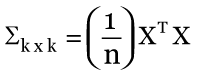Where,

k = number of stocks in the portfolio

n = number of observations

X = this is the n x k excess return matrix. We will understand this better shortly

XT  = transpose matrix of X

Here is a quick explanation of what is going on in that formula. You may understand this better when we deal with its implementation.

In simple terms, we first calculate the n x k excess return matrix; multiply this matrix by its own transpose matrix. This is a matrix multiplication and the resulting matrix will be a k x k matrix. We then divide each element of this k x k matrix by n, where n denotes the number of observations. The resulting matrix after this division is a k x k variance covariance matrix.

Generating the k x k variance covariance matrix is one step away from our final objective i.e getting the correlation matrix.

So, let us apply this formula and generate the variance covariance matrix for the 5 stocks listed above. I’m using MS excel for this. I have downloaded the daily closing prices for the 5 stocks for the last 6 months.

Step 1 – Calculated the daily returns. I guess you are quite familiar with this by now. I’m not going to explain how to calculate the daily returns. Here is the excel snapshot.As you can see, I’ve lined up the stock’s closing price and next to it I have calculated the daily returns. I have indicated the formula to calculate the daily return.

Step 2 – Calculate the average daily returns for each stock. You can do this by using the ‘average’ function in excel.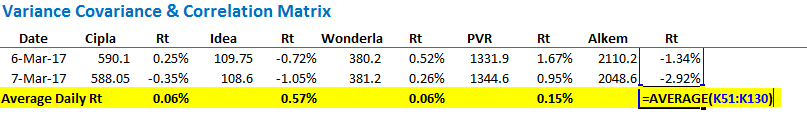Step 3 – Set up the excess return matrix.

Excess return matrix is defined as the difference between stock’s daily return over its average return. If you recall, we did this in the previous chapter while discussing covariance between two stocks.

I’ve set up the excess return matrix in the following way –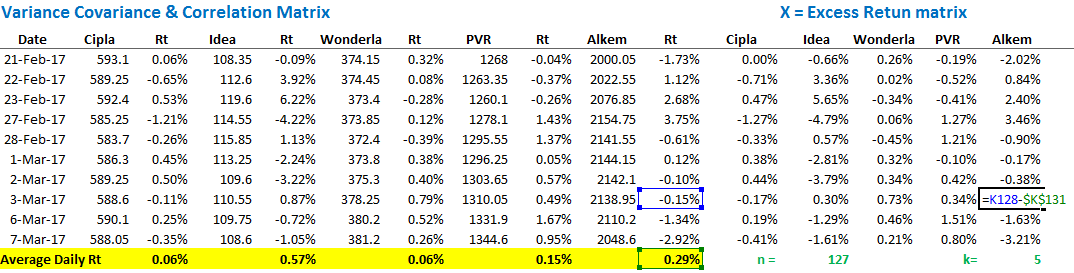Do note, the resulting matrix is of n x k size, where n represents the number of observations (127 in this case) and k denotes the number of stocks (5 stocks). So in our example the matrix size is 127 x 5. We have denoted this matrix as X.

Step 4 – Generate the XT X matrix operation to create a k x k matrix

This may sound fancy, but it is not.

XT is a new matrix, formed by interchanging the rows and columns of the original matrix X. When you interchange the rows and columns of a matrix to form a new one, then it is referred to as a transpose matrix of X and denoted as XT. Our objective now is to multiply the original matrix with its transpose. This is denoted as XT X.

Note, the resulting matrix from this operation will result in a k x k matrix, where k denotes the number of stocks in the portfolio. In our case this will be 5 x 5.

We can do this in one shot in excel. I will use the following function steps to create the k x k matrix –

List down the stocks in rows and columns –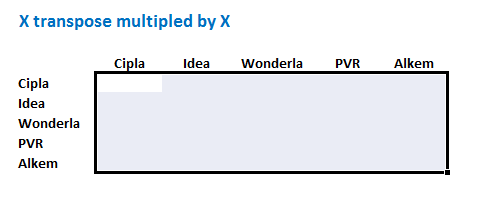Apply the function = ‘MMULT ((transpose X), X). Remember X is the excess return matrix.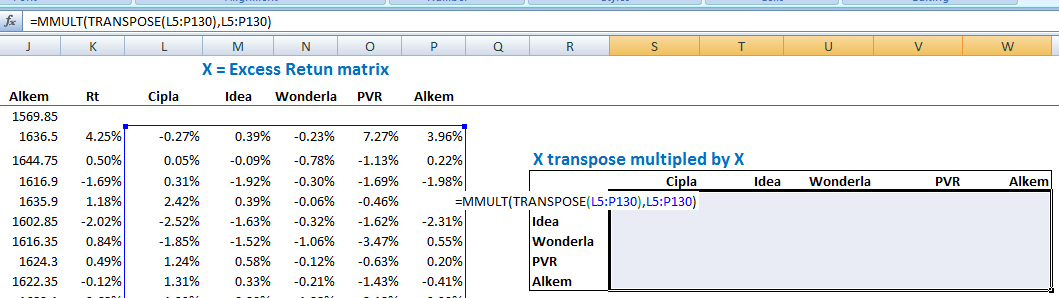Do note, while applying this formula, you need to ensure that you highlight the k x k matrix. Once you finish typing the formula, do note – you cannot hit ‘enter’ directly. You will hit ctrl+shift+enter. In fact, for all array functions in excel, use ctrl+shift+enter.

So once you hit ctrl+shift+enter, excel will present you with a beautiful k x k matrix, which in this case looks like this –Step 5 – This is the last step in creating the variance covariance matrix. We now have to divide each element of the XT X matrix by the total number of observations i.e n. For your clarity, let me post the formula for the variance covariance matrix again –Again, we start by creating the layout for k x k matrix –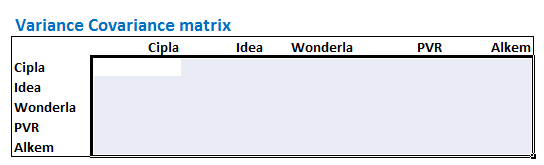Once the layout is set, without deselecting the cells, select the entire XT X matrix and divide it by n i.e 127. Do note, this is still an array function; hence you need to hit ctrl+shift+enter and not just enter.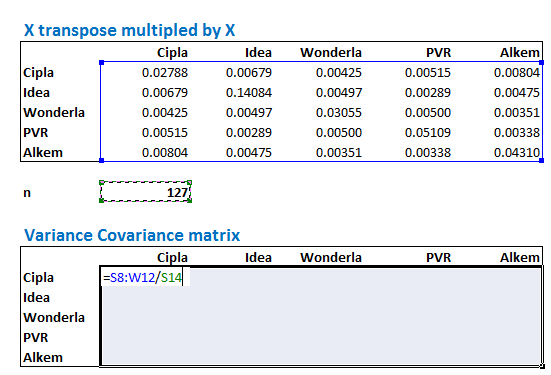Once you hit control shift enter, you will get the ‘Variance – Covariance’ matrix. Do note, the numbers in the matrix will be very small, do not worry about this. Here is the variance co variance matrix –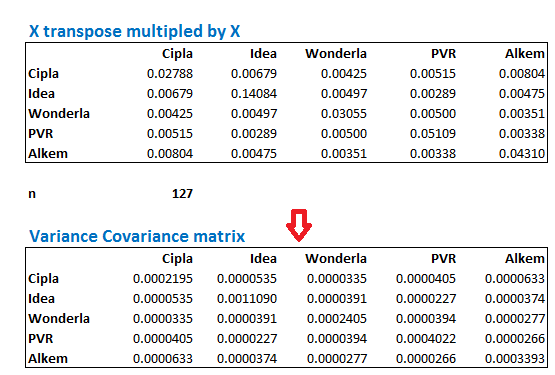Let us spend some time to understand the ‘Variance – Covariance’ matrix better. Suppose I want to know the covariance between any two stocks, lets say Wonderla and PVR, then I simply have to look for Wonderla on the left hand side and in the same row, look for the value which coincides with PVR. This would be the covariance between the two stocks. I’ve highlighted the same in yellow –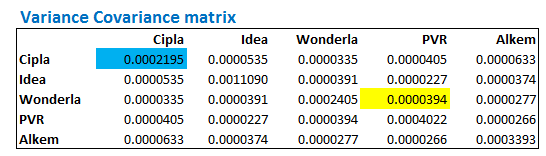So the matrix suggests that the covariance between Wonderla and PVR is 0.000034. Do note, this is the same as the covariance between PVR and Wonderla.

Further, notice the number highlighted in blue. This value corresponds to Cipla and Cipla. What do you this represents? This represents the covariance between Cipla and Cipla, and if you realize, covariance of a stock with itself, is nothing but variance!

This is exactly why this matrix is called ‘Variance – Covariance Matrix’, cause it gives us both the values.

Now, here is the bitter pill – the variance and covariance matrix on its own is quite useless. These are extremely small numbers and it is hard to derive any meaning out of it. What we really need is the ‘Correlation Matrix’.

In the next chapter, let us deal with generating the correlation matrix, and also work towards estimating the portfolio variance, which is our end objective. However, before we close this chapter, here are few tasks for you –

1. Download the last 1 year data for 5 or more stocks.
2. Calculate the Variance – Covariance matrix for the same
3. For a given stock, identify the variance value. Apply the = ‘Var()’ function on excel on the returns of the same stock and evaluate if both are matching.

### Key Takeaways from this chapter

1. X is defined as an excess return matrix
2. Excess return matrix is simply the time series difference daily return versus the average daily return
3. XT is defined as the transpose of X
4. Variable n is defined as the number of observations in the data set. For example if you have 6 months data, n is 127, for 1 year data n would be 252
5. Excess return matrix is of the size n x k, where k is the number of stocks
6. When you divide the matrix product of XT X by n, we get the variance covariance matrix
7. The variance covariance matrix is of the size k x k
8. The covariance of stock 1 with itself is the variance of stock 1
9. The variance covariance matrix will lead us to the correlation matrix.
Module 9

#### Chapters

1.bbhalaji says:

Hi Karthik, before we start building a portfolio, is it a good idea to make the co variance analysis explained in this chapter using the sectoral indices and then pick sectors that have a negative covariance. Then we can pick stocks from these selected sectors ?? this is just to make sure that I am handling the unsystematic risk even before I pick the stock. Thanks.

•Karthik Rangappa says:

No, always pick stocks based on factors such as business prospect, numbers, margins etc. Once you have a portfolio, you can go ahead and calculate these things,….remember, we are gearing towards finding out the portfolio risk. That will eventually help us asset allocation etc. I think the next chapter or 2 will give you some insights into this.

2.Naresh says:

Hi, Karthik, Just slightly unclear about corrrelation and Beta. U explained that correlation gives you the magnitude of a relationship between 2 stocks and I guess Beta which we use in futures hedging also did the same thing ie. magnitude of relationship between stock and index. Can you add few words to simplify and explain the subtle differences and commonalities between Beta and Corelation. Thanks

•Karthik Rangappa says:

Beta is basically a measure to estimate the market risk of a stock (systemic risk), in a sense, it is a measure or risk…also unlike correlation, beta is not bound by values. Correlation on the other had defines how two variables move and are related.

3.akashbarman says:

Dear Sir , this is really an impressive write up . I have browsed through hundreds of sites to quench my thirst for the stock markets but with no success. Finally i landed up at Varsity n its great for a beginner like up..
I have been waiting for a write up on Financial Modelling topic . It would be great if u educate us on the same .

•Karthik Rangappa says:

It is one of the topics that I like too 🙂

Will take it up sometime soon.

4.jayshil says:

very informative!! Sir, will you please explain correlation/std dev between two stocks? Also some knowledge about pair trading..

5.vj says:

•Karthik Rangappa says:

No, its useful if you have a long term portfolio.

6.Santosh Shetti says:

Hi Karthik,
Desperately waiting for your next chapter. Thanks for informing us about screener.in. It’s a great site fully loaded with financial data of Indian Companies.
Just one query, suppose market value is 10 times the book value what does it indicate? How much difference/ratio is acceptable to take Investment call?

Thanks & regards

•Karthik Rangappa says:

Will try and put the chapter by end of this week or early next week. 10 time BV means that the stock is trading 10 time BV 🙂

It gives an idea on how cheap or expensive the stock is from a valuation perspective. You need to compare stocks in the same sector to identify how cheap or expensive the stock really is.

7.Kapil Sharma says:

Hi Karthik,

Thanks for these very informative and helpful articles. My questions about calculating variance co-variance matrix is – How do I choose number of observations? What should be my base date to start calculating daily returns given the date of purchase of different stocks will vary.

Thanks!

•Karthik Rangappa says:

Kapil, the number of observations is at your discretion. It could be 6 months, 1 year, 2 years or even 5. The starting date for return calculation is always the 2 date from the start as the formula required you to divide today’s return over yesterday’s.

•Kapil Sharma says:

Thanks Karthik for the prompt response. From your answer, I am getting the impression that date of investment doesn’t matter for this calculation. May be I will get more clarity once you explain calculating the correlation and applying that value for portfolio risk analysis. Eagerly waiting for next chapter. Best!

•Karthik Rangappa says:

I guess the next chapter will be available tomorrow.

8.sandeep says:

good work sir
thank you .

•Karthik Rangappa says:

Cheers.

9.Anil Nagesh says:

Thanks Karthik for Such brilliant material for variance and co variance. I have one query Up to Excess matrix i got the solution, While doing the transpose matrix. I am getting same value of all stocks. Because formula for transpose matrix is same for all.

•Karthik Rangappa says:

The values will remain the same, the order of the transpose matrix changes.

10.Aman kabra says:

Sir, in excel you have taken the average daily return as per following calculation =AVERAGE(C51:C130). but as I understand from the above chapter, all observations should be considered for average calculation. Please explain.

•Karthik Rangappa says:

Hey, Amam…looks like that’s a mistake. Thanks for pointing that out. I guess the whole mode will change slightly based on this one silly mistake. But the general steps would be the same.

11.Arun Mahalingam says:

Hi Karthik,

In step 2 of your explanation, I think the idea is to calculate average return for the complete time period (n). The formula here and in the spreadsheet calculates average only from row 51 to 130 – should it not be from row 5 to 130?

When I tried doing the portfolio variance calculation by variance-covariance matrix and compare it with the method given in next chapter, there seems to be a slight difference – but the numbers exactly match in your example (however, with that glitch of row 51 to 130 instead of 5 to 130). Was it tweaked intentionally for any specific reason?

Rgdz,
Arun

•Arun Mahalingam says:

Sorry, I just see the post from Amam. But the question that continues is how can the variance numbers match for you when you do it with the other method? How can it be 1.11% in both cases?

•Karthik Rangappa says:

The difference could be beyond the 2nd decimal I guess.

•Karthik Rangappa says:

Yes, Arun. Looks like that’s a mistake. You just have to extend the selection to all rows. Naturally, the numbers will change.

12.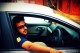Himanshu Singhal says:

I think the values calculated are wrong here.
I have a screenshot of the error but I will try to explain it here.
The very first thing is that the average taken of each individual stocks is wrong.

Example: if we look at the excel as shared in this varsity (downloadable attachment) shows average of stocks as thus
Cipla in Cell C 131 = 0.06%, Idea in cell E131 = 0.57%, Wonderla in cell G131 = 0.06%, PVR in cell I131 = 0.15% and Alkem in cell K131 = 0.29%.

However, actual values upon calculation are Cell C 131 = 0.02%, cell E131 = 0.26%, cell G131 = -0.05%, cell I131 = 0.12% and cell K131 = 0.23%.

*The error was in calculating the average at the first step i.e for example for Cipla = average(C51:C131) instead should be average(C5:C131).
The same for all the other 4 stocks.

Hope you will ratify the error and repost.

•Karthik Rangappa says:

Absolutely agree, Himanshu. I’ve missed calculating the average for all cells but by mistake calculated only for few cells. Someone pointed this earlier as well (check the comments). I; ve not really rectified this as these calculations would have to be changed across many chapters. I regret this mistake.

However, I’d suggest you download the excel and make that small correction, the model will self-correct.

•Himanshu says:

I have already worked on the correct values, it seems like there is alot of variance when it comes to solver for Portfolio Optimization.
One of the stock goes negative thus making the margin between Portfolio Return & Variance marginal.

Kindly look into this.

•Karthik Rangappa says:

Ah, maybe I’ll have to rework on this.

•Himanshu says:

Do you want me to send you the xls?

•Karthik Rangappa says:

Can you? [email protected]. Thanks.

•Himanshu says:

Yes I have shared the file. Also I have a doubt,
When we are calculating the Excess returns (through variance Covariance VaR method);
The columns containing excess returns of the 5 stocks from L to P (xls) have x-mu.
But when we calculate variance we do ( ∑ 〖(X−mu)〗^2 )/n.
Here we neither add all the value nor square the values, although the method claims variance-covariance method.
Could you please explain the doubt?

•Karthik Rangappa says:

X Transpose X matrix takes care of this, Himanshu.

13.Brahma Reddy says:

In your “X Transpose Multiplied by X Matrix” each cell contains five values after its decimal point.But in the matrix which is calculated by me contains eight values after decimal point.My actual problem while calculating variance-covariance matrix by dividing by number of observations,all the cells are showing zero sir.Pls help me.

•Karthik Rangappa says:

I have a feeling you are missing doing the Control+shift+enter, can you please confirm? Thanks.

•Brahma Reddy says:

No sir,Iam using them right.But same problem persists.Help me sir.Thank u

•Karthik Rangappa says:

Ah, unfortunately, I won’t be able to figure out until I see the excel.

•Manda Brahma Reddy says:

mail id pls sir.I will send it.

•Karthik Rangappa says:

[email protected]

•Brahma Reddy says:

I have send you the mail sir.

•Karthik Rangappa says:

To which email id?

•Brahma Reddy says:

•Karthik Rangappa says:

Will do, thanks.

•Brahma Reddy says:

The ticket number given for my request was Ticket#20170927790025

•Karthik Rangappa says:

Thanks, will follow up with my team on Tuesday.

•Brahma Reddy says:

•Karthik Rangappa says:

Will do.

•Brahma Reddy says:

•Karthik Rangappa says:

Will try and do that next week.

14.Himanshu says:

Hi Karthik,

I was working on the same model for HCC stock, I found that my min value for retrun was -89.0%.
Later I found that till 7-Nov-05 the price was at 994.3 and the next day on 9-Nov-05 the price was 99.3.
I believe it might be some sort of stock split or something.
If yes, then could you explain how to use VaR model using Histrogram with a stock that has stock splits in between.

Thanks.

•Karthik Rangappa says:

If there is a stock split/bonus then you really have to adjust the price historically to reflect the split/bonus. This is the only way to go about.

15.Narasimha says:

I have checked the mutual funds portfolio sir.In asset allocation they gave heavy weightage to banking/finance sector.Any reason ?? Is it better to follow while building a individual portfolio ?

•Karthik Rangappa says:

It really depends on the MF scheme and the fund manager’s call.

16.Harsh says:

Instead of comparing variance and covariance with each stock why not take a common benchmark like nifty or sensex and compare its covariance with it and choose stocks ?

•Karthik Rangappa says:

When you have stocks in the portfolio, there is also risk of co-movements of the stock. This risk is quantified by the variance-covariance matrix.

17.Ajay says:

Hi,

How come the excess matrix in excel sheet is (n-1) * K matrix right ?
you have assumed n as 127 and divided by 127.. should it be 126 by any chance ?

either excess matrix size is (n-1)*k or n is 126.. which one is right ?

•Karthik Rangappa says:

Ah, its n-1. Guess I’ve made a mistake.

18.Priya says:

Hi Kartik,
I have been in equities from past 6 months. Unknowingly i have purchased lot of scrips and now my portfolio consists of around 82 Scrips. now if i want to calculate variance covariance matrix for entire portfolio how can i do that? from where can i get the details of the closing price data. i have been maintaining my portfolio on moneycontrol. is there any alternate to calculate for such a huge number of scrips?

Kindly Help.

•Karthik Rangappa says:

Priya, even before you get into this calculation, I’d suggest you consolidate your portfolio. 82 is a bit too much. I have 18 in my portfolio and I’m very uncomfortable with it. I guess something around 12-15 is perfect.

Unfortunately, there is no alternative here. Maybe you could try programming this if you know how to program.

•Priya says:

Will these 12 -15 scripts include long term shares which we buy and forget?

•Karthik Rangappa says:

Yes, it should.

19.Arun says:

Hello sir,
I just had a confusion about the variance part. The variance calculated by the the formula for every stock exceeds by exactly 22.22% of the value from the matrix. I tried to find possible steps I might have messed up, but came up with nothing. So I did the thing that as an engineer I can do; I wittingly reduced the value of ‘n’. And solved the problem and saved the day.?
So, what I wanted to ask you is this. Is it necessary for the number of observations has to be a big number to get the exact value of variance in the matrix?

•Karthik Rangappa says:

Arun, I’m sorry…I’m unable to get that 22.22% part. Anyway, ideally the look-back period should be at least 1 year (252 trading days) for this.

•Arun says:

Sir, the deviation(as percentage of ‘=VAR’ value) between the values of variance from the “=VAR()” function and the Var-Cov Matrix were 22.22%. And I checked it with the provided worksheet, after correcting the averages. There too was a minor variation. The thing is, when I put the value of ‘n’ as ‘n-2’ in the formula of VC matrix, there was no deviation anymore. So, is it ok to replace the value of n by n-2??

•Karthik Rangappa says:

Hmmm, Ideally it should be n-1. If n is large, then n-2 should not be very different from n-1 I guess.

20.Diptiranjan says:

Hi Karthik,

Please guide me to about stock selection for portfolio. Is it available in any where in versity?

regards,
Diptiranjan

•Karthik Rangappa says:
21.edwen says:

How did you get 127 as n

•Karthik Rangappa says:

N is the number of data points.

22.Simranjeet Singh says:

Dear Karthik sir,
I observed in your excel sheet of this chapter, While calculating Average daily returns of each stock, you only took the average of “Cell 51 to Cell 130 i.e Average Daily Rt =AVERAGE(C51:C130). Kindly elaborate this. This is a confusing point because i think you should take averages of all cells from “C5 to C130”. Afterall this alters the whole result of analysis.

•Karthik Rangappa says:

Yes, Simranjeet, I’m aware of this and have discussed this in various comments. I think I missed dragging the selection to the entire column. Since I have used these values across multiple chapters, fixing it across is a nightmare. However, the calculation method remains the same.

•Simranjeet Singh says:

•Karthik Rangappa says:

Good luck!

23.nitesh gode says:

have a query..is this usefull for traders for who hold stocks for 1,2 or 3 weeks or a month….i dont like to hold stocks more than some weeks

•Karthik Rangappa says:

Not really, Nitesh. More applicable to longer-term time frame.

24.Arindam says:

Dear sir

I calculate variance covariance matrix for 14 shares mentioned below as per method explained in the module. But the result I got at the end is numbers in most of the cases with letter E in it as below.Except in variance of same share to share like astral to astral, bajajfin to bjajfin and so on I get numbers. Otherwise there is letter E in it.

Is it in order? or there is something wrong in it? what does this E signifies?

regards
arindam

VARIANCE COVARIANCE MATRIX

Astral Bajajfin HDFC ICICIGI bajajfinserv kotakbank Marico Pageind Pidilite Relaxo AsianPanin Bergerpaint ITC Lalpathlab
Astral 0.000478888896107 5.53920841704367E-05 -3.1354792812574E-06 3.03007935680207E-05 4.88356389420704E-05 2.24562133988781E-05 4.31141464629457E-05 4.93499070229815E-05 4.73097331821573E-05 2.31779218587669E-05 2.88167953405164E-05 -6.4786094655855E-06 1.80785027032291E-05 3.17602448194354E-05
bajaj 5.53920841704367E-05 0.000463529531102 2.74506824398392E-06 9.71739643987807E-05 0.000317632814914 2.70337830273591E-05 6.2562581904229E-05 9.44418782951298E-05 1.80615271964162E-07 4.34070375604452E-05 1.95866168910395E-05 1.78168112533641E-05 7.04755911788722E-05 2.49726408520599E-05
hdfc -3.1354792812574E-06 2.74506824398392E-06 0.000249566453938 9.24628492088152E-06 -1.09450457624853E-05 -1.92744382577237E-05 -1.70961923123606E-05 1.60024262597867E-05 -4.6259657480803E-06 8.46229340842743E-06 3.03015176738522E-06 -1.43059924528607E-05 8.53503726551041E-06 -1.24242081359671E-05
icicgi 3.03007935680207E-05 9.71739643987807E-05 9.24628492088152E-06 0.000399701820618 8.6710267957033E-05 6.35034848707978E-07 1.26263404097151E-05 3.84156469087817E-05 -9.47463523687206E-06 1.49652145379937E-05 -3.90628052986236E-06 -3.68906701398572E-06 2.45217245392575E-05 1.50401911983464E-05
bajajfinsev 4.88356389420704E-05 0.000317632814914 -1.09450457624853E-05 8.6710267957033E-05 0.000367248940012 3.21082388893008E-05 7.40728359724138E-05 8.27767796038017E-05 3.45837453510943E-06 1.03650595573159E-05 1.23664881452636E-06 -7.42523676666824E-06 7.4942540527155E-05 2.09994610280924E-05
kotakbank 2.24562133988781E-05 2.70337830273591E-05 -1.92744382577237E-05 6.35034848707978E-07 3.21082388893008E-05 0.000219124966998 2.08614027619164E-05 9.23224378081341E-06 -2.909759976866E-06 -5.57679786485266E-06 8.10579490816851E-06 1.10922020620547E-06 1.23999709211708E-06 -8.59999939608236E-06
marico 4.31141464629457E-05 6.2562581904229E-05 -1.70961923123606E-05 1.26263404097151E-05 7.40728359724138E-05 2.08614027619164E-05 0.000210319839648 4.25657771817425E-05 2.64094187120521E-05 -2.35677429608113E-05 3.41762515471651E-06 2.02900748683665E-06 2.36057795976331E-05 -3.66629152360838E-06
pageind 4.93499070229815E-05 9.44418782951298E-05 1.60024262597867E-05 3.84156469087817E-05 8.27767796038017E-05 9.23224378081341E-06 4.25657771817425E-05 0.000574946287503 8.71999400314927E-06 4.15256436284941E-05 1.75225376198826E-05 5.93448667239259E-05 1.66711350994658E-05 7.92197744913677E-05
pidilite 4.73097331821573E-05 1.80615271964162E-07 -4.6259657480803E-06 -9.47463523687206E-06 3.45837453510943E-06 -2.909759976866E-06 2.64094187120521E-05 8.71999400314927E-06 0.000264275057675 4.03222542290343E-05 6.3246188110903E-05 0.000131957339917 1.7071268466552E-05 2.46030602909579E-05
relaxo 2.31779218587669E-05 4.34070375604452E-05 8.46229340842743E-06 1.49652145379937E-05 1.03650595573159E-05 -5.57679786485266E-06 -2.35677429608113E-05 4.15256436284941E-05 4.03222542290343E-05 0.000730080568759 3.80289064137802E-05 8.25664245182325E-05 6.08337318124509E-06 1.12482824171446E-05
asian paint 2.88167953405164E-05 1.95866168910395E-05 3.03015176738522E-06 -3.90628052986236E-06 1.23664881452636E-06 8.10579490816851E-06 3.41762515471651E-06 1.75225376198826E-05 6.3246188110903E-05 3.80289064137802E-05 0.000211504715438 0.000107906225212 2.03522936293495E-05 3.83155015325739E-05
berger paint -6.4786094655855E-06 1.78168112533641E-05 -1.43059924528607E-05 -3.68906701398572E-06 -7.42523676666824E-06 1.10922020620547E-06 2.02900748683665E-06 5.93448667239259E-05 0.000131957339917 8.25664245182325E-05 0.000107906225212 0.000342151390903 8.52098814103089E-06 3.16432289394699E-05
itc 1.80785027032291E-05 7.04755911788722E-05 8.53503726551041E-06 2.45217245392575E-05 7.4942540527155E-05 1.23999709211708E-06 2.36057795976331E-05 1.66711350994658E-05 1.7071268466552E-05 6.08337318124509E-06 2.03522936293495E-05 8.52098814103089E-06 0.000167574112566 -1.3428901458124E-05
lalpath 3.17602448194354E-05 2.49726408520599E-05 -1.24242081359671E-05 1.50401911983464E-05 2.09994610280924E-05 -8.59999939608236E-06 -3.66629152360838E-06 7.92197744913677E-05 2.46030602909579E-05 1.12482824171446E-05 3.83155015325739E-05 3.16432289394699E-05 -1.3428901458124E-05 0.000385211183891

•Karthik Rangappa says:

Its is a very small number, you can approximate it to the nearest decimal.

15 chapters

21 chapters

16 chapters

12 chapters

23 chapters

13 chapters

7 chapters

19 chapters

16 chapters